Math & Beyond

# Alphabet Test for Kids!

2.4k views

 1 Introduction 2 Best Practises for solving the problems on the Alphabetical Test 3 Example Questions 4 Practice Questions 5 Conclusion 6 Frequently Asked Questions (FAQs)

23 December 2020

## Introduction to Alphabets

Mental aptitude and reasoning tests cover many topics, the list is quite long. Among all topics, the alphabet test is one of the crucial topics. It covers questions depending on the alphabetical order, misplacing of the alphabets, etc.

Alphabets are the very first thing that kids learn in schools. The different alphabets with different shapes are the first doorstep of knowledge to kids.

Kids try to remember all these shapes right from A to Z, with its pronunciation and pictures. After understanding the alphabet, they start their journey with words and sentences.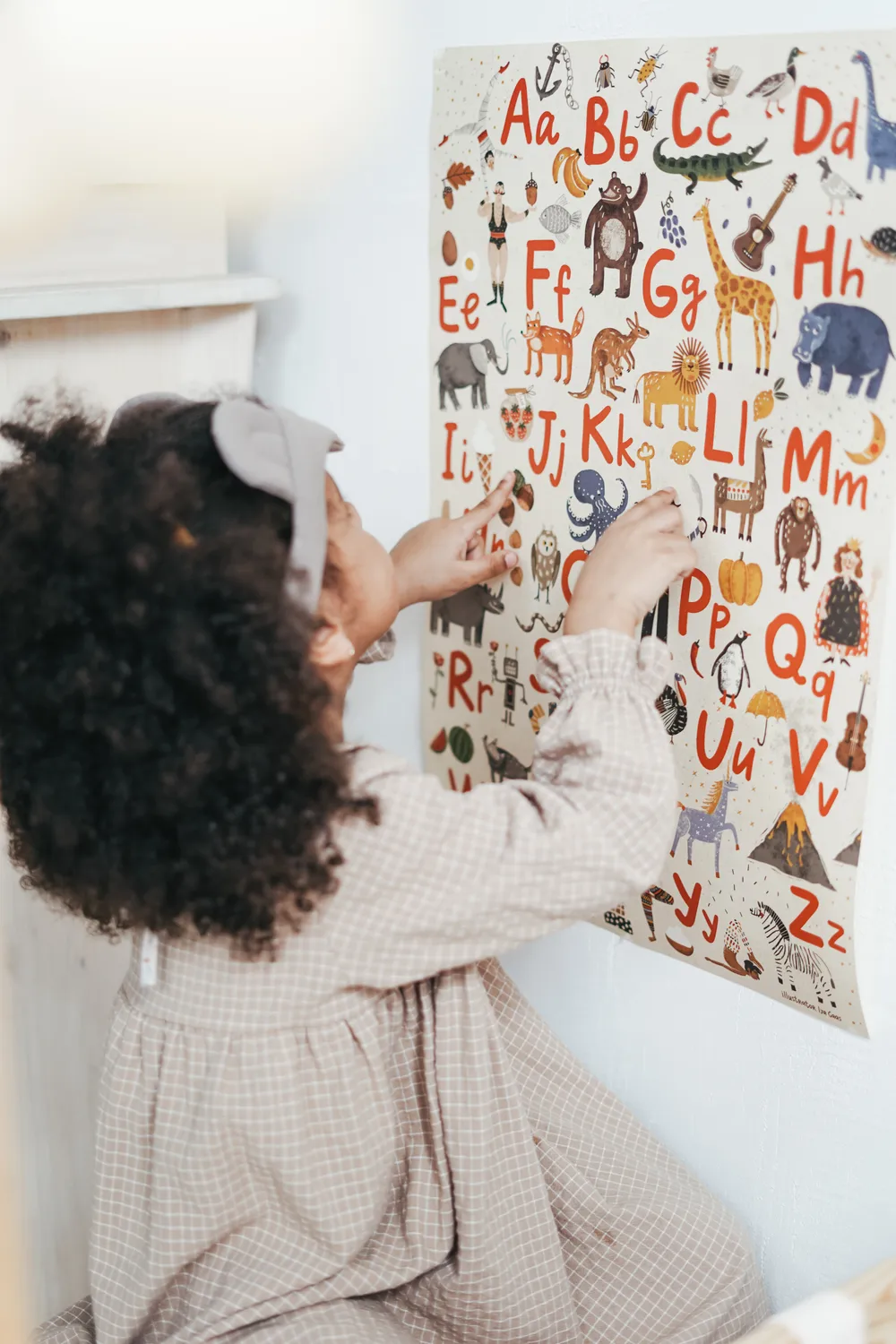These alphabets also play a vital role when the students want to prepare for their reasoning and mental ability in higher grades. So, the alphabet test is a very important topic.

There are different types of question patterns based on these alphabet tests such as finding out the place of an alphabet, or a word based on the different types of arrangements of alphabets, there are some questions of alphabetical arrangements where we have to arrange words in alphabetical order, some questions are about rearrangement of letters to form meaningful words, etc.

We can classify all types of questions discussed above as follows for better understanding.

• Rearrange letters or numbers to form a meaningful word
•  Find the word that can/cannot be formed from the given letters.
• Form a meaningful word and identify an appropriate category
• Identify the combination of letters that has the same number of skipped letters between the adjacent letters
• Find the exact alphabet according to the given conditions.
• Arrange the given words in alphabetical orders.
• Sequencing of alphabets

## Alphabet Test for Kids!

Alphabet Test is one of the easiest and important concepts of the General Mental Ability Segment of Reasoning. In this type of question, students are asked to find the place of an alphabet or a word based on the different types of arrangement.

## Best Practises for solving the problems on the Alphabetical Test

For solving the questions in alphabetical order, one should follow the following practices for exact and quick results.

1. Observe the alphabets given in each question.
2. Try to find the pattern or sequence it follows.
3. Decipher the code assigned to each alphabet.
4. Figure out the rule followed by the given arrangement of alphabets/words.
5. Always refer to the given options to get a sense of the answer to be found.

## Example Questions

### Rearrange letters or numbers to form a meaningful words

Question: Some letters are given which are numbered 1, 2, 3, 4, 5, and 6 followed by four options containing combinations of these numbers. Find the combination such that letters when arranged accordingly form meaningful words.

G    S    D    N    E    I

1    2     3    4    5   6

(A) 314562- DGNEIS   (B) 364125- DINGSE   (C) 352614-DESIGN   (D) 534621-EDNISG

 (C) 352614-DESIGN

### Find the word that can/cannot be formed from the given letters

Question: Find the word which CANNOT be made using the letters of the word given.

(A) MIND    (B) RATIO    (C) MINISTER    (D) STATION

Answer:  Observing the given options, we can conclude that the MINISTER word cannot be formed by the ADMINISTRATION  word, as it does not contain the letter  E ''. So option (C)  is the answer.

 (C) MINISTER

### Form a meaningful word and identify an appropriate category

Question: Find the category of the word formed by rearranging the letters to form a meaningful word.

RTNEIW
(A) Fruit    (B) Vegetable    (C) Season    (D) Flower

Answer:  RTNEIW word rearranges to form the word WINTER, which is Season. So option (C) is the answer.

 (C) Season

### Identify the combination of letters that has the same number of skipped letters between the adjacent letters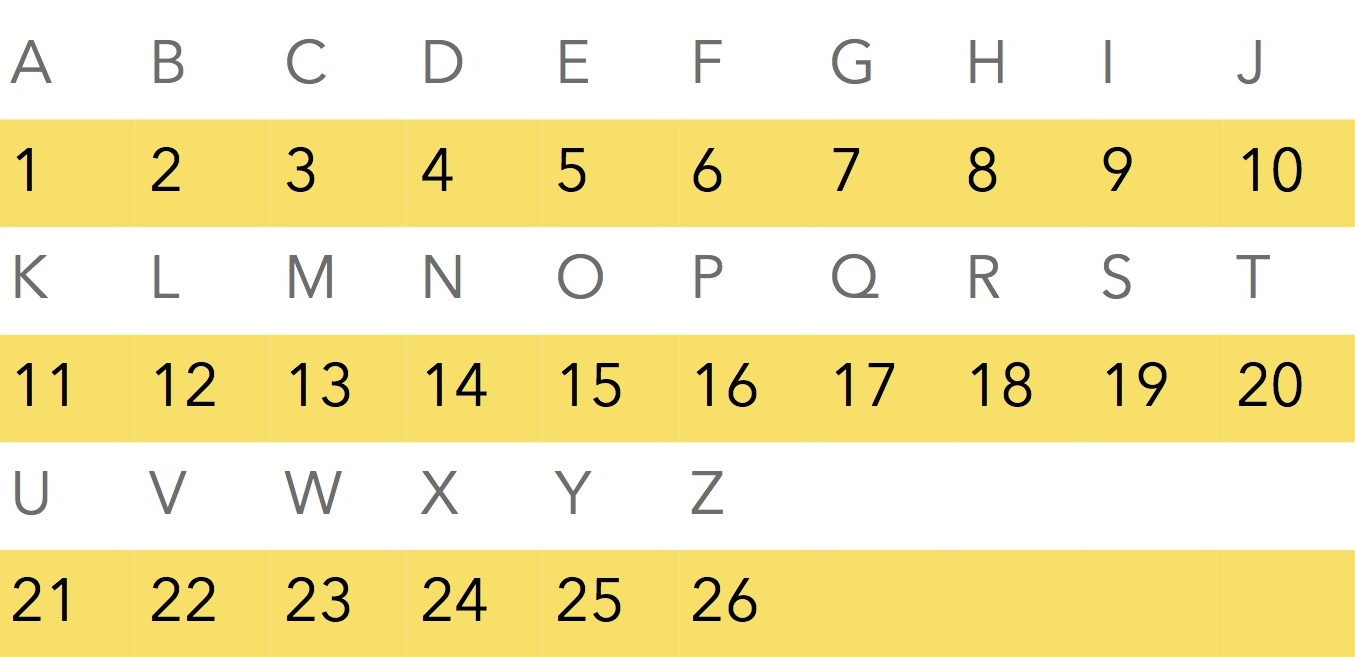Question: Which series follows the rule given?

Rule: The number of letters skipped in between adjacent letters in the series decreases by two.
(A) FQWBG    (B) TBINO    (C) HQXCF    (D) XFMQU

Answer:  We noticed that in HQXCF, the alphabet H and Q has 8 alphabets in between them which go on decreasing by 2. So, there are 6 alphabets between Q and X, there are 4 alphabets between X and C and 2 alphabets between C and F. So as per the given rule option (C) is the answer.

 (C) HQXCF

### Find the exact alphabet according to the given conditions

Question: Find the 7th letter to the left of the 21st letter from the left in the English alphabet.

(A)  D    (B) J    (C) N    (D) I

Answer:  We can easily find out the 21st letter is U and the   7th letter to the left of U is N, therefore option ( C) N is the answer.

 (C) N

### Arrange the given words in alphabetical orders

Question: What should come in the fifth place of question marks (?) in the following letter series based on the English alphabetical order?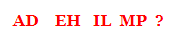(A) SU    (B) QT    (C) QS    (D) None of these

Answer:  We can say that there are two letters between A and D, E and H, I and L, M and P, so next set of letters in the series would be QT, so option (B) is the answer.

 (B) QT

## Practice Questions

1. Some letters are given which are numbered 1, 2, 3, 4, 5, and 6 followed by four options containing combinations of these numbers. Find the combination such that letters when arranged accordingly form meaningful words.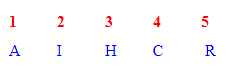(A) 52134   (B) 43125   (C) 21435   (D) 54321

2. Find the word which CAN be made using the letters of the word given.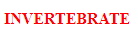(A) Investment     (B) Revert      (C) Entrance    (D) None of These

3. Find the category of the word formed by rearranging the letters to form a meaningful word.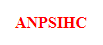(A) Vegetable         (B) Season     (C) Flower      (D) None of These

4. Which series follows the rule given?
Rule: The number of letters skipped in between adjacent letters in the series decreases by   two.
Find the 10th letter to the left of the 15th letter from the left in the English alphabet.

(A)  X             (B) J       (C) N        (D) E

5. Arrange these words in alphabetical order and tick the one that comes second last.
1. Oscillate    2. Orphanage    3. Outburst    4. Ostensible    5. Outrage

(A) Oscillate     (B) Outburst  (C) Ostensible   ( D) None of the these

 1. A         2. B       3. A     4. D     5. B

## Conclusion

Alphabets test is a very vast topic, we can generate several possibilities and questions depend on this. The above mentioned questions are the most asked in the aptitude examination.

It is very necessary to follow the exact procedure to solve these questions in less time which can be definitely achieved by regular practice.

-Written by Uttara Varade, Cuemath Teacher

Cuemath, a student-friendly mathematics and coding platform, conducts regular Online Live Classes for academics and skill-development, and their Mental Math App, on both iOS and Android, is a one-stop solution for kids to develop multiple skills. Understand the Cuemath Fee structure and sign up for a free trial.

## What is alphabetic series?

In Alphabet Series section, a string of alphabets, either in a single file or in combination form a sequence.

## How do you solve the alphabet test?

Basic Concepts & Tricks to Alphabet Test

1. Place of letter in forward order.
2. Place Of Letter in Completely Backward Order.
3. Place of a Letter When First Half is in Backward Order.
4. Place of a Letter When Second Half is in Backward Order.
5. Multiple Letter Segment in Backward Order.
6. Number of Letter in the Middle of Two Letters.

Related Articles
GIVE YOUR CHILD THE CUEMATH EDGE
Access Personalised Math learning through interactive worksheets, gamified concepts and grade-wise courses